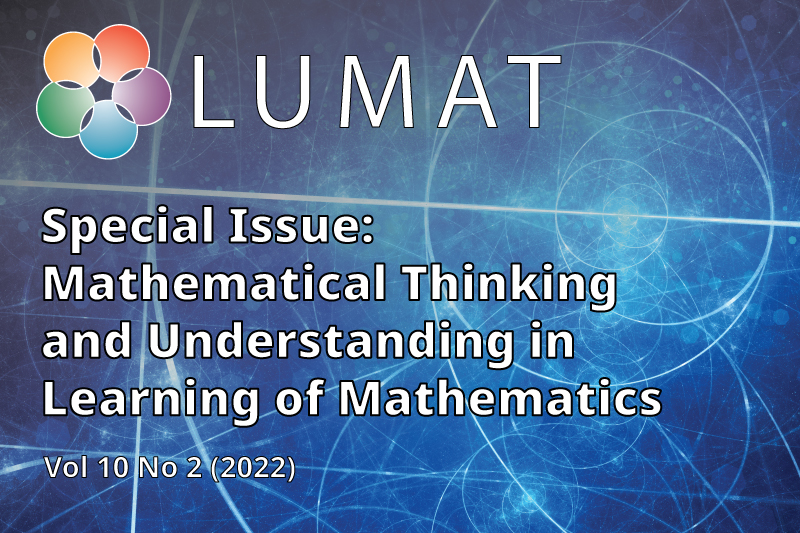# Vol. 10 No. 2 (2022): Mathematical Thinking and Understanding in Learning of MathematicsThe concept of mathematical thinking is traditional and has been interpreted over decades or even centuries according to the prevailing notions of each era. When, we have focus on the learning process the concept mathematical thinking can be approached from several different directions: mathematical concepts, how express mathematical thinking, developing mathematical thinking by manipulatives (concrete or digital), developing mathematical thinking at different ages and many other points of view. The Special Issue consists of eleven articles about mathematical thinking and the role of understanding in mathematics learning from the interesting points of view.

Guest editors
Jorma Joutsenlahti, Tampere University, Finland
Päivi Perkkilä, Kokkola University Consortium Chydenius, University of Jyväskylä, Finland

Published: 2022-06-30

## Foreword

• ### Mathematical thinking and understanding in learning of mathematics

Jorma Joutsenlahti, Päivi Perkkilä
1–6

## What is mathematical thinking?

• ### The three worlds and two sides of mathematics and a visual construction for a continuous nowhere differentiable function

Juha Oikkonen, Jani Hannula
7–32

33–55
• ### Rudimentary stages of the mathematical thinking and proficiency Mathematical skills of low-performing pupils at the beginning of the first grade

Jari Metsämuuronen, Annette Ukkola
56–83

## How can we express mathematical thinking?

• ### Preschoolers’ ways of experiencing numbers

Camilla Björklund, Anna-Lena Ekdahl, Angelika Kullberg, Maria Reis
84–110
• ### Developing mathematical problem-solving skills in primary school by using visual representations on heuristics

Susanna Kaitera, Sari Harmoinen
111–146
• ### Supporting argumentation in mathematics classrooms The role of teachers’ mathematical knowledge

John Francisco
147–170
• ### Languaging and conceptual understanding in engineering mathematics

Kirsi-Maria Rinneheimo, Sami Suhonen
171–189

## Conceptual understanding and mathematical thinking

• ### Identifying and promoting young students’ early algebraic thinking

Sanna Wettergren
190–214
• ### “Learning models” Utilising young students’ algebraic thinking about equations

Inger Eriksson, Natalia Tabachnikova
215–238
• ### Understanding “proportion” and mathematical identity A study of Japanese elementary school teachers

Kazuyuki Kambara
239–255
• ### Student teachers’ common content knowledge for solving routine fraction tasks

Anne Tossavainen
256–280